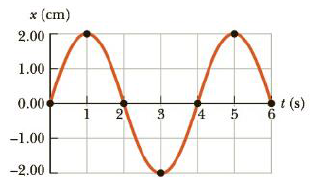Chapter 13, Problem 42P

Chapter
Section
Textbook Problem

An object attached to a spring vibrates with simple harmonic motion as described by Figure P13.42. For this motion, find (a) the amplitude, (b) the period, (c) the angular frequency, (d) the maximum speed, (e) the maximum acceleration, and (f) an equation for its position x in terms of a sine function.Figure P13.42

(a)

To determine
The amplitude of simple harmonic motion.

Explanation

Amplitude: Amplitude A   is defined as the maximum displacement of the object from the equilibrium position.

The harmonic motion is represented in the graph below.

From the figure, maximum displacement of the object from equilibrium position is 2

b)

To determine
The period of the simple harmonic motion.

c)

To determine
The angular frequency of the simple harmonic motion.

d)

To determine
The maximum velocity of the simple harmonic motion.

e)

To determine
The maximum acceleration of the simple harmonic motion.

f)

To determine
An equation for position x in terms of sine function.

Still sussing out bartleby?

Check out a sample textbook solution.

See a sample solution

The Solution to Your Study Problems

Bartleby provides explanations to thousands of textbook problems written by our experts, many with advanced degrees!

Get Started

Find more solutions based on key concepts